# 18.5: Decarboxylation of Carboxylic Acids

$$\newcommand{\vecs}{\overset { \rightharpoonup} {\mathbf{#1}} }$$ $$\newcommand{\vecd}{\overset{-\!-\!\rightharpoonup}{\vphantom{a}\smash {#1}}}$$$$\newcommand{\id}{\mathrm{id}}$$ $$\newcommand{\Span}{\mathrm{span}}$$ $$\newcommand{\kernel}{\mathrm{null}\,}$$ $$\newcommand{\range}{\mathrm{range}\,}$$ $$\newcommand{\RealPart}{\mathrm{Re}}$$ $$\newcommand{\ImaginaryPart}{\mathrm{Im}}$$ $$\newcommand{\Argument}{\mathrm{Arg}}$$ $$\newcommand{\norm}{\| #1 \|}$$ $$\newcommand{\inner}{\langle #1, #2 \rangle}$$ $$\newcommand{\Span}{\mathrm{span}}$$ $$\newcommand{\id}{\mathrm{id}}$$ $$\newcommand{\Span}{\mathrm{span}}$$ $$\newcommand{\kernel}{\mathrm{null}\,}$$ $$\newcommand{\range}{\mathrm{range}\,}$$ $$\newcommand{\RealPart}{\mathrm{Re}}$$ $$\newcommand{\ImaginaryPart}{\mathrm{Im}}$$ $$\newcommand{\Argument}{\mathrm{Arg}}$$ $$\newcommand{\norm}{\| #1 \|}$$ $$\newcommand{\inner}{\langle #1, #2 \rangle}$$ $$\newcommand{\Span}{\mathrm{span}}$$

The decarboxylation of $$\ce{RCO_2H}$$ to give $$\ce{RH}$$ and $$\ce{CO_2}$$ can be calculated from bond energies and the stabilization energy of the carboxyl group to have $$\Delta H^0 = -7 \: \text{kcal mol}^{-1}$$. This does not mean that the reaction goes easily. Special structural features are required. The simple aliphatic carboxylic acids do not lose carbon dioxide on heating, but when there are strongly electron-attracting groups attached to the $$\alpha$$ carbon, decarboxylation often proceeds readily at $$100$$-$$150^\text{o}$$. Examples include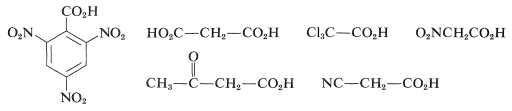3-Butenoic acid also undergoes decarboxylation but has to be heated to above $$200^\text{o}$$:The mechanisms of thermal decarboxylation probably are not the same for all cases, but when the acid has a double-bonded function such as $$\ce{O=C}$$, $$\ce{N=C}$$, $$\ce{O=N}$$, or $$\ce{C=C}$$ attached to the $$\alpha$$ carbon then a cyclic elimination process appears to occur. For propanedioic acid the process is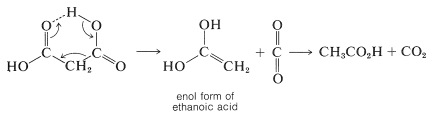Carboxylate radicals can be generated in several ways. One is the thermal decomposition of diacyl peroxides, which are compounds with rather weak $$\ce{O-O}$$ bonds: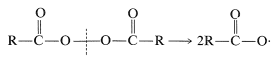Another method involves electrolysis of sodium or potassium carboxylate solutions, known as Kolbe electrolysis, in which carboxylate radicals are formed by transfer of an electron from the carboxylate ion to the anode. Decarboxylation may occur simultaneously with, or subsequent to, the formation of carboxylate radicals, leading to hydrocarbon radicals, which subsequently dimerize:An example isDecarboxylation of the silver salts of carboxylic acids in the presence of bromine or chlorine, the Hunsdiecker reaction, often is useful for the synthesis of alkyl halides: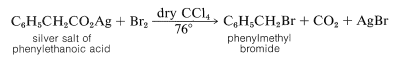The mechanism of this reaction seems to involve formation of carboxylate radicals through decomposition of an acyl hypobromic intermediate, $$12$$:The Hunsdiecker reaction has certain disadvantages, mainly because it requires use of the pure dry silver salt, which is often difficult to prepare. With some acids, however, excellent results can be obtained using the acid itself and an excess of red mercuric oxide in place of the silver salt.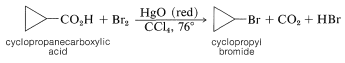or by heating the acid with lead tetraethanoate, $$\ce{Pb(O_2CCH_3)_4}$$, and iodine,A somewhat similar decarboxylation reaction with formation of an alkene can be achieved by heating a carboxylic acid with lead tetraethanoate, $$\ce{Pb(O_2CCH_3)_4}$$, in the presence of a catalytic amount of $$\ce{Cu(OCH_3)_2}$$. A useful example isThere is some competing decarboxylation of the ethanoic acid, but the conversions in this kind of reaction are usually good. The key steps in the reaction probably are exchange of carboxylic acid groups on tetravalent lead, cleavage of the $$\ce{Pb-O}$$ bond to give the carboxylate radical, decarboxylation, oxidation of the alkyl radical by $$\ce{Cu}$$ (II) to give the cation $$\left[ \ce{R} \cdot + \ce{Cu} (II) \rightarrow \ce{R}^\oplus + \ce{Cu} (I) \right]$$, and finally loss of a proton to form the alkene.